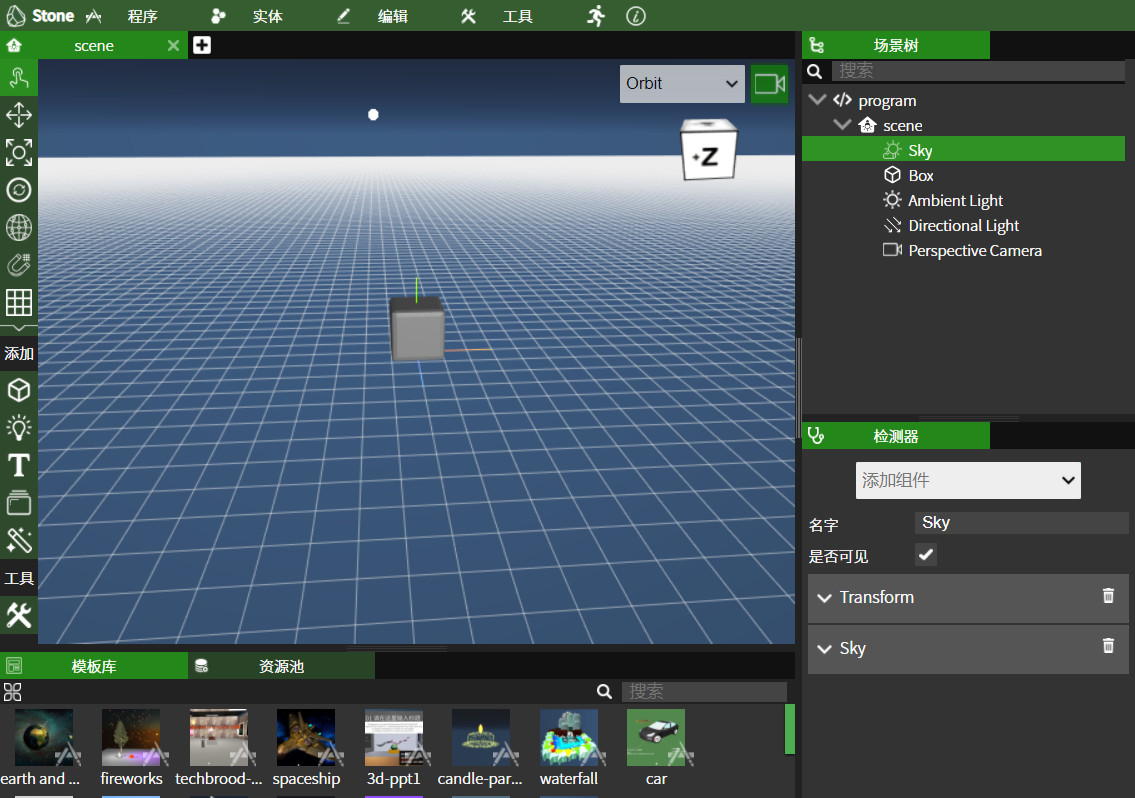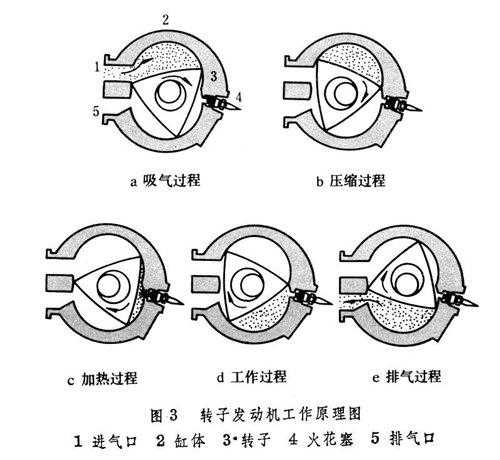## 电脑技术：来自B站的 Manim 教程合集

Manim教程合集

B站的这些视频来自YouTube，https://www.youtube.com/watch?v=ENMyFGmq5OA。“想知道3Blue1Brown大佬的数学视频是如何制作出来的么？这里是一套Manim的教程合集，完结撒花！”

# Kinect and Processing

The Microsoft Kinect sensor is a peripheral device (designed for XBox and windows PCs) that functions much like a webcam. However, in addition to providing an RGB image, it also provides a depth map. Meaning for every pixel seen by the sensor, the Kinect measures distance from the sensor. This makes a variety of computer vision problems like background removal, blob detection, and more easy and fun!

The Kinect sensor itself only measures color and depth. However, once that information is on your computer, lots more can be done like “skeleton” tracking (i.e. detecting a model of a person and tracking his/her movements). To do skeleton tracking you’ll need to use Thomas Lengling’s windows-only Kinect v2 processing libray. However, if you’re on a Mac and all you want is raw data from the Kinect, you are in luck! This library uses libfreenect and libfreenect2 open source drivers to access that data for Mac OS X (windows support coming soon).

## 电脑技术+建模+艺术：一个很牛的沉浸式交互网站——踏得网## 知识：转子发动机1 油耗高，污染重。转子发动机的转子运动特点是：三角转子的中心绕输出轴中心公转的同时，三角转子本身又绕其中心自转。在三角转子转动时，以三角转子中心为中心的内齿圈与以输出轴中心为中心的齿轮啮合，齿轮固定在缸体上不转动，内齿圈与齿轮的齿数之比为3:2。上述运动关系使得三角转子顶角的运动轨迹（即汽缸壁的形状）似“8”字形。三角转子利用转子的顶角把汽缸分成三个独立空间，三个空间各自先后完成进气、压缩、做功和排气，三角转子自转一周，发动机点火做功三次。这样大家明白了转子的运动关系，其输出轴的转速是转子自转速度的3倍，这与往复运动式发动机的活塞与曲轴是1:1的运动关系完全不同。由于没有往复式发动机的高压缩比，使得燃烧不能够很充分。虽然马自达公司曾经给转子发动机增加了单涡轮增压和双涡轮增压等东西，但只是提高了输出马力，并适度的减少了尾气排放，但还是与往复式发动机有着很大的差距。

2 零部件寿命短由于转子的三个顶角负责密封（顶角上也有类似活塞环一样的密封件），并且长期处于无法良好润滑的情况下工作，导致其过早的磨损。虽有很多种方案进行了改进，例如将气缸壁进行瓷化（对气缸壁表面进行陶瓷化，可以减少摩擦，并且在无润滑的情况下更耐高温），对于三个顶角的密封材料进行筛选，等等，但始终无法有更好的突破。并且转子中间的输出轴部位的高温下问题，始终困扰着工程师们。

3 利益传统生产往复式发动机的厂家们，对于往复式发动机已经投入了大量的财力人力物力去改造去完善去创新了，在往复式发动机的潜力还没有挖掘尽的情况下，转而投入财力人力物力去改造前景不是很光明的转子发动机，我想，每个往复式发动机厂家的CEO都会摇头的。况且，还有各种环保法规的出台，也预示着转子发动机的末日。尤其是该死的1992年，在1991年马自达夺得勒芒24小时汽车赛冠军后，被国际汽联明令禁止参赛，其背后利益的关联，可想而知了。

## 用MATHEMATICA计算一元高次方程（续）

StartYear是起始年份，EndYear是结束年份，Year是一共经历了多少年。

StartNumber 就是初始年份的值，EndNumber是结束年份的值。## 用Mathematica的 DSolve 解微分方程时返回True的原因和解决办法

Question: DSolve returns true

Using Mathematica 7, I got a solution

The only time I was able to reproduce the “DSolve[True….” message was when I had the sequence of commands as follows,

What’s happening is that y'[x] is known to be x- y[x], and the DSolve is doing a compare of y-y[x] == y-y[x], returning True. Then it can’t solve True for y[x] in x, resulting in the question being returned.

Would you try exiting the kernel, restart fresh, and re-typing (* commands 1*) only.

（内容出自 Wolfram Community http://community.wolfram.com/groups/-/m/t/332674

# 控件对象

Wolfram 语言提供一系列完整的控件对象，所有对象以便捷的符号形式表示. Manipulate 自动调用这些对象；您也可以在一般输入时直接调用它们，或在您自己的动态界面内调用它们.

Control 一个自动选择类型的交互控制

Slider, VerticalSliderSlider2DCheckboxRadioButtonOpenerIntervalSlider 使用下界和上界设值

ColorSlider 交互选择一个颜色

Setter 设置按下鼠标时的值:Toggler 点击时切换值

PopupMenu 选择菜单

ListPicker 选项列表

InputField 可接受字符串、数字或任何表达式的输入栏

FormControl 含有释义明确的字段的任意表单

LocatorPane 图形或其它背景上可移动的定位器

Button 可以设置任意外观和行为的按钮

PasteButton 复制表达式的按钮

ActionMenu, ButtonBar 选择行为的菜单

EventHandler 低层次事件处理

FileNameSetter 系统文件浏览

ColorSetter 系统颜色选择

SystemDialogInput 文件、字体、声音等

Hyperlink 超链接到单元和网址

FormObject 指定格式结构

FormFunction 设置活动的可提交格式

# 相关教程

## 计算张量的软件

EDC and RGTC，即 Riemannian Geometry & Tensor Calculus @ Mathematica，链接：http://www.inp.demokritos.gr/~sbonano/RGTC/

## Download RGTC (Version 3.8.9 – May 2013)

• Download all files – compressed: .sit format (100 KB), .zip format (135 KB)
• Uncompressed files (~1000 KB):  RGTC.nb  —  OperatorPLT.nb  —  NPsymbolPLT.nb  —  EDCRGTCcode.m. (Only the combined matrixEDC and RGTC code in package format is included — it must be placed in an appropriate directory).
• Note: RGTC cannot be used for calculations with abstract tensors (manipulation of tensor expressions with abstract indices). It only operates on explicit tensors (nested lists of components which are functions of the coordinates). For abstract calculations try the package xTensor.

Additional Examples can be found here.

## Standalone software

• SPLATT is an open source software package for high-performance sparse tensor factorization. SPLATT ships a stand-alone executable, C/C++ library, and Octave/MATLABAPI.
• Cadabra is a computer algebra system (CAS) designed specifically for the solution of problems encountered in field theory. It has extensive functionality for tensor polynomial simplification including multi-term symmetries, fermions and anti-commuting variables, Clifford algebras and Fierz transformations, implicit coordinate dependence, multiple index types and many more. The input format is a subset of TeX. Both a command-line and a graphical interface are available.
• Tela is a software package similar to Matlab and (GNU) Octave, but designed specifically for tensors.

## Software for use with Mathematica

• Tensor is a tensor package written for the Mathematica system. It provides many functions relevant for General Relativity calculations in general Riemann-Cartan geometries.
• Ricci is a system for Mathematica 2.x and later for doing basic tensor analysis, available for free.
• TTC Tools of Tensor Calculus is a Mathematica package for doing tensor and exterior calculus on differentiable manifolds.
• EDC and RGTC, “Exterior Differential Calculus” and “Riemannian Geometry & Tensor Calculus,” are free Mathematica packages for tensor calculus especially designed but not only for general relativity.
• Tensorial “Tensorial 4.0” is a general purpose tensor calculus package for Mathematica.
• xAct: Efficient Tensor Computer Algebra for Mathematica. xAct is a collection of packages for fast manipulation of tensor expressions.
• GREAT is a free package for Mathematica that computes the Christoffel connection and the basic tensors of General Relativity from a given metric tensor.
• Atlas 2 for Mathematica is a powerful Mathematica toolbox which allows to do a wide range of modern differential geometry calculations
• GRTensorM is a computer algebra package for performing calculations in the general area of differential geometry.
• MathGR is a package to manipulate tensor and GR calculations with either abstract or explicit indices, simplify tensors with permutational symmetries, decompose tensors from abstract indices to partially or completely explicit indices and convert partial derivatives into total derivatives.
• TensoriaCalc is a tensor calculus package written for Mathematica 9 and higher, aimed at providing user-friendly functionality and a smooth consistency with the Mathematica language itself. As of January 2015, given a metric and the coordinates used, TensoriaCalc can compute Christoffel symbols, the Riemann curvature tensor, and Ricci tensor/scalar; it allows for user-defined tensors and is able to perform basic operations such as taking the covariant derivatives of tensors. TensoriaCalc is continuously under development due to time constraints faced by its inventor/developer.

## Software for use with Maple

• GRTensorII is a computer algebra package for performing calculations in the general area of differential geometry.
• Atlas 2 for Maple is a modern differential geometry for Maple.
• DifferentialGeometry is a package which performs fundamental operations of calculus on manifolds, differential geometry, tensor calculus, General Relativity, Lie algebras, Lie groups, transformation groups, jet spaces, and the variational calculus. It is included with Maple.

## Software for use with Maxima

Maxima is a free open source general purpose computer algebra system which includes several packages for tensor algebra calculations in its core distribution. It is particularly useful for calculations with abstract tensors, i.e., when one wishes to do calculations without defining all components of the tensor explicitly. It comes with three tensor packages:

• itensor for abstract (indicial) tensor manipulation,
• ctensor for component-defined tensors, and
• atensor for algebraic tensor manipulation.

## Software for use with R

• Tensor is an R package for basic tensor operations.
• rTensor provides several tensor decomposition approaches.
• tensorBF is an R package for Bayesian Tensor decomposition.
• MTF Bayesian Multi-Tensor Factorization for data fusion and Bayesian versions of Tensor PCA and Tensor CCA. Software: MTF

## Libraries

• Redberry is an open source computer algebra system designed for symbolic tensor manipulation. Redberry provides common tools for expression manipulation, generalized on tensorial objects, as well as tensor-specific features: indices symmetries, LaTeX-style input, natural dummy indices handling, multiple index types etc. The HEP package includes tools for Feynman diagrams calculation: Dirac and SU(N) algebra, Levi-Civita simplifications, tools for calculation of one-loop counterterms etc. Redberry is written in Java and provides extensive Groovy-based programming language.
• libxm is a lightweight distributed-parallel tensor library written in C.
• FTensor is a high performance tensor library written in C++.
• TL is a multi-threaded tensor library implemented in C++ used in Dynare++. The library allows for folded/unfolded, dense/sparse tensor representations, general ranks (symmetries). The library implements Faa Di Bruno formula and is adaptive to available memory. Dynare++ is a standalone package solving higher order Taylor approximations to equilibria of non-linear stochastic models with rational expectations.
• vmmlib is a C++ linear algebra library that supports 3-way tensors, emphasizing computation and manipulation of several tensor decompositions.
• Spartns is a Sparse Tensor framework for Common Lisp.
• FAstMat is a thread-safe general tensor algebra library written in C++ and specially designed for FEM/FVM/BEM/FDM element/edge wise computations.
• Cyclops Tensor Framework  is a distributed memory library for efficient decomposition of tensors of arbitrary type and parallel MPI+OpenMP execution of tensor contractions/functions.
• TiledArray is a scalable, block-sparse tensor library that is designed to aid in rapid composition of high-performance algebraic tensor equation. It is designed to scale from a single multicore computer to a massively-parallel, distributed-memory system.
• libtensor  is a set of performance linear tensor algebra routines for large tensors found in post-Hartree-Fock methods in quantum chemistry.
• ITensor  features automatic contraction of matching tensor indices. It is written in C++ and has higher-level features for quantum physics algorithms based on tensor networks.
• Fastor  is a high performance C++ tensor algebra library that supports tensors of any arbitrary dimensions and all their possible contraction and permutation thereof. It employs compile-time graph search optimisations to find the optimal contraction sequence between arbitrary number of tensors in a network. It has high level domain specific features for solving nonlinear multiphysics problem using FEM.
• Xerus  is a C++ tensor algebra library for tensors of arbitrary dimensions and tensor decomposition into general tensor networks (focusing on matrix product states). It offers Einstein notation like syntax and optimizes the contraction order of any network of tensors at runtime so that dimensions need not be fixed at compile-time.